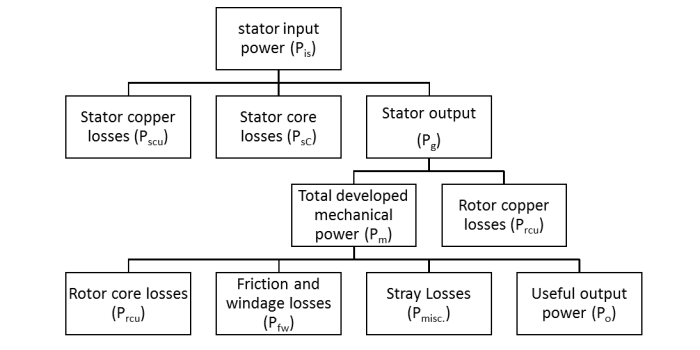# Power Flow Diagram and Losses of Induction Motor

The 3-phase input power fed to the stator of a 3-phase induction motor is given by,

$$\mathrm{𝑃_{𝑖𝑠} = \sqrt{3} 𝑉_𝐿𝐼_𝐿 cos \varphi_i = 3 𝑉_{𝑠𝑝ℎ} 𝐼_{𝑠𝑝ℎ} cos \varphi_i}$$

Where,

• VL = Line voltage
• IL = Line current
• Vsph = Stator phase voltage
• Isph = Stator phase current
• cos \varphii = input power factor

## Stator Losses

• Stator copper losses or I2R losses in the stator winding resistances, which are given as follows −

$$\mathrm{𝑃_{𝑠𝑐𝑢} = 3 𝐼_{𝑠𝑝ℎ}^{2} 𝑅_{𝑠𝑝ℎ}}$$

• Hysteresis and eddy current losses in the stator core, which are known as stator-core losses and are given by −

$$\mathrm{𝑃_{𝑠𝐶} = 𝑃_{𝑠ℎ} + 𝑃_{𝑠𝑒}}$$

Therefore, the power output of the stator will be,

$$\mathrm{𝑃_{𝑜𝑠} = 𝑃_{𝑖𝑠}− 𝑃_{𝑠𝑐𝑢} − 𝑃_{𝑠𝐶}}$$

This output power of the stator (Pos) is transferred to the rotor of the machine across the air-gap between the stator and the rotor. It is also known as air-gap power (Pg) of the machine. Hence,

$$\mathrm{Power\:output\:of\:stator (𝑃_{𝑜𝑠})\:=\: Air\:gap\:power (𝑃_𝑔)\:=\:Input\:power\:to\:rotor (𝑃_{𝑖𝑟})}$$

## Rotor Losses

• Rotor copper losses or I^2R losses in the rotor resistance, which are given by,
• $$\mathrm{𝑃_{𝑟𝑐𝑢} = 3 𝐼_2^2 𝑅_2}$$

• Rotor core losses or hysteresis and eddy current losses, which are given as follows −
• $$\mathrm{𝑃_{𝑟𝐶} = 𝑃_{𝑟ℎ} + 𝑃_{𝑟𝑒}}$$

• Friction and windage losses (Pfw)
• Stray load losses (Pmisc) consisting of all the losses which are not included in the above losses like losses due to harmonic fields.

## Mechanical Power Developed (Pm)

If the rotor copper losses are subtracted from the rotor input power (Pg or Pir), then the remaining power is converted from electrical power to mechanical power. It is known as developed mechanical power (Pm).

$$\mathrm{Developed\:mechanical\:power,\: 𝑃_𝑚 = 𝑃_{𝑖𝑟} − 𝑃_{𝑟𝑐𝑢}}$$

$$\mathrm{⇒ 𝑃_𝑚 = 𝑃_𝑔 − 𝑃_{𝑟𝑐𝑢} = 𝑃_𝑔 − (3𝐼_2^2𝑅_2)}$$

Therefore, the output power of the motor is given by,

$$\mathrm{𝑃_𝑜 = 𝑃_𝑚 − 𝑃_{𝑓𝑤} − 𝑃_{𝑚𝑖𝑠𝑐}}$$

The power Po is known as useful power or shaft power.

## Rotational Losses

At the starting and during the acceleration, the rotor core losses are high and decreases with the increase in the speed of the motor. The friction and windage losses are zero at start and increases with the increases in the speed.

Consequently, the sum of core losses and friction and windage losses is approximately constant with varying speed of the motor. Hence, these losses may lumped together and are called as rotational losses and given as follows −

$$\mathrm{𝑃{𝑟𝑜𝑡𝑎𝑡𝑖𝑜𝑛𝑎𝑙\:𝑙𝑜𝑠𝑠𝑒𝑠}= 𝑃_𝐶 + 𝑃_{𝑓𝑤} + 𝑃_{𝑚𝑖𝑠𝑐}}$$

Then, the output power of the motor is given by,

$$\mathrm{𝑃_𝑜 = 𝑃_𝑚 − 𝑃{𝑟𝑜𝑡𝑎𝑡𝑖𝑜𝑛𝑎𝑙\:𝑙𝑜𝑠𝑠𝑒𝑠}= 𝑃_𝑜 = 𝑃_𝑚 − 𝑃_𝐶 − 𝑃_{𝑓𝑤} − 𝑃_{𝑚𝑖𝑠𝑐}}$$

The power diagram of the induction motor is shown in the figure below.